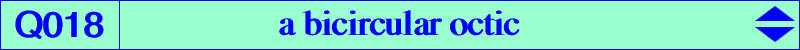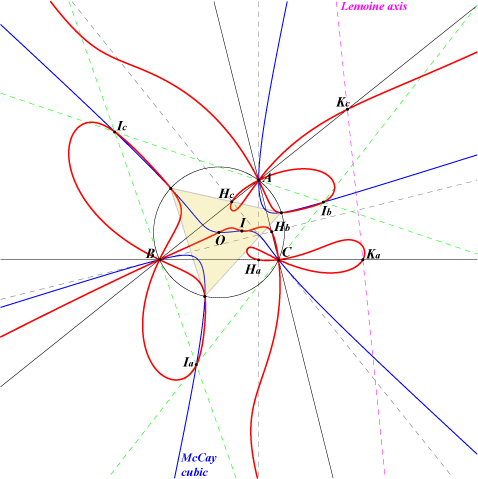too complicated to be written here. Click on the link to download a text file.X(1), X(3), X(2574), X(2575) A, B, C (triple) Ia, Ib, Ic : excenters Ha, Hb, Hc : feet of altitudes Ka, Kb, Kc : feet of the Lemoine axis (centers of the apollonian circles) X(2574), X(2575) : points at infinity of the Jerabek hyperbola vertices of the circumnormal triangle (on the McCay cubic)Let P be a point and let H', H" be the orthocenters of the pedal and antipedal triangles of P respectively. P, H', H" are collinear if and only if P lies on the octic Q018 or on the line at infinity. Q018 has five singular points : A, B, C (triple) and the circular points at infinity (double). The tangents at A are the line AO and two other perpendicular lines passing through the intercepts of the circle centered at Oa = AO /\ BC through A and BC. Q018 has two real asymptotes which are parallel to those of the Jerabek hyperbola. It meets the circumcircle at the vertices of the circumnormal triangle.